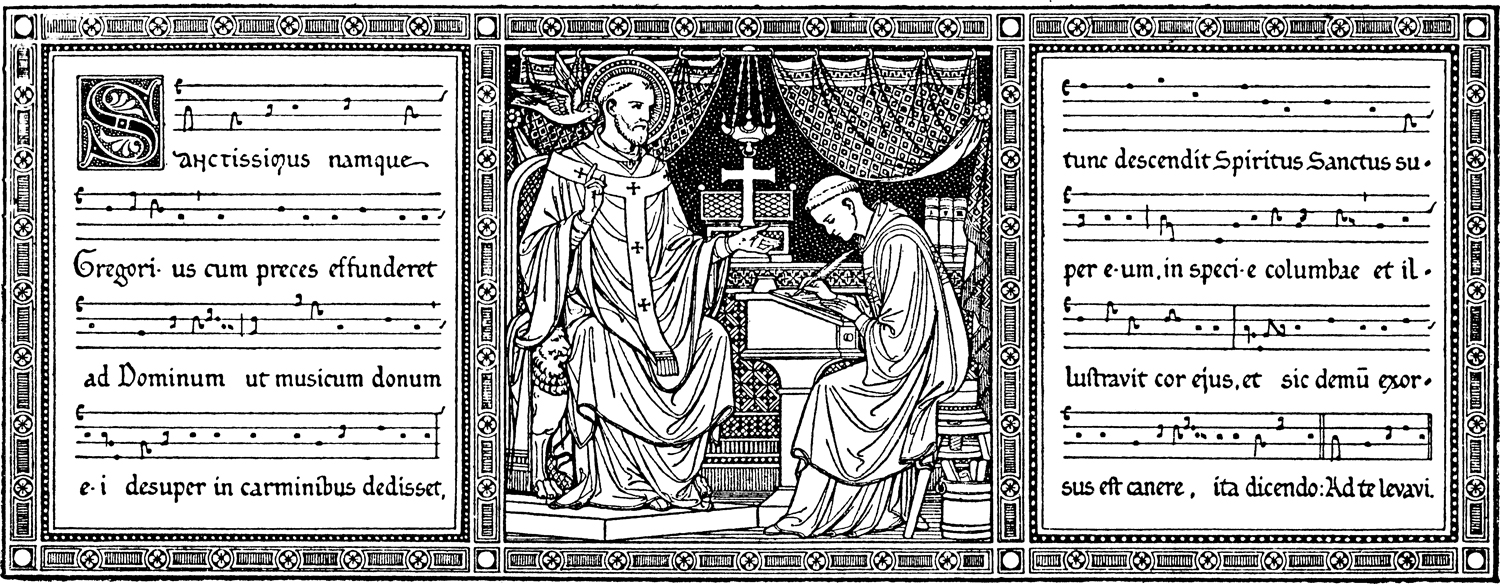(Subtitle: another neat thing I picked up from the MusicaSacra Forum)

It’s too bad I don’t have a category called “Lore”; this would have to go in it.  At the beginning  of the 1908 (Vatican) Graduale we have this picture:Which is actually not just a picture, but a singable chant score!

This trope from the 11th-12th Century was sung in some form before the Introit of the First Sunday of Advent. It replaced an earlier trope for this Introit honoring St. Gregory, which had been composed by Pope Hadrian I (772 – 795), which was placed at the top of the Sacramentary given by Pope Hadrian to Charlemagne, the existence of which was attested to by Agobard of Lyons (d. 840).

Latin: Sanctíssimus namque Gregórius cum preces effúnderet ad Dóminum ut músicum donum ei désuper in carmínibus dedísset, tunc descéndit Spíritus Sanctus super eum, in spécie colúmbæ, et illustrávit cor ejus, et sic demum exórtus est cánere, ita dicéndo : Ad te levavi… (& we continue the Introit)

English: When the most holy Gregory poured out prayers to the Lord that He might surrender to him from above a musical gift in song then the Holy Spirit descended upon him in the form of a dove and enlightened his heart to such a degree that at last he began to sing saying thus: “Ad te levavi…” (& we continue the Introit) (tr. Katherine Bergeron)

A recording of the chant can be heard here.  The introit which follows, Ad te levavi, is significant because being that of the First Sunday of Advent, it is thus the first piece of chant in the Graduale.  Hence, it is the first thing which the inspired Gregory would have dictated.

Here are two transcriptions I did from the illustration and recording, for anyone who now feels compelled to have handy a performing edition of Sanctissimus namque Gregorius:

I. As seen above

II. With Solesmes rhythmic markings

You can consider singing it before the introit on the First Sunday of Advent this year!

From → Gregorian Chant

1.Is it possible to have this Trope Sanctissimus, in a gabc version ?

2.Here is the gabc code I had saved from when I made the scores; I would guess that it should be correct, but you may want to check it.

Sanctissimus namque Gregorius

name:;
%%
(c4)SAn(gd~)ctís(fe)si(fg)mus(g) nam(gh)que(gf) Gre(h)gó(hj)ri(ih)us(g) (,) cum(g) pre(h)ces(g) ef(g)fún(h)de(g)ret(g) ad(g) Dó(f)mi(gh)num(hg/hi/h/gg) (;) ut(fh) mú(jk)si(ji)cum(g) do(h)num(g) e(g)i(gvFD) dé(fe)su(fg)per(g) in(g) car(g)mí(g)ni(f)bus(gh) de(h)dís(g)set,(g) (:) tunc(j) des(j)cén(k)dit(i) Spí(j)ri(h)tus(g) San(h)ctus(g) su(fe)per(fg) e(g)um,(g) (;) in(gfd~) spé(d)ci(f)e(g) co(hg)lúm(f!gh)bæ,(hgg) (,) et(f) il(g)lus(h)trá(ji)vit(hg) cor(hih) e(g)jus,(g) (:) et(gvFDfhfg) sic(g) de(h)mum(g) e(g)xór(h)tus(g) est(g) cá(f)ne(gh)re,(hg/hi/h/gg) i(g)ta(g) di(gf)cén(hi)do:(g) (::) Ad(gd~) te(f) le(gh)va(h)vi.(g) (:)

With ictus, etc.

name:;
%%
(c4)SAn(gd~)ctís(fe)si(fg)mus(g) *() nam(gh)que(gf) Gre(h)gó(hj)ri(ih)us(g.) (,) cum(g) pre(h)ces(g’) ef(g)fún(h’)de(g)ret(g’) ad(g) Dó(f)mi(gh)num(hg/hiHG’g) (;) ut(fh) mú(jk)si(ji)cum(g) do(h)num(g’) e(g)i(gvFD) dé(fe)su(fg)per(g.) (,) in(g) car(g)mí(g’)ni(f)bus(gh) de(h)dís(g.)set,(g.) (:) tunc(j) des(j’)cén(k)dit(i’) Spí(j)ri(h)tus(g) San(h’)ctus(g) su(fe)per(fg) e(g)um,(g.) (;) in(gf) spé(d)ci(f’)e(g) co(hg)lúm(f!gh)bæ,(hg..) (,) et(f) il(g’)lus(h)trá(ji)vit(hg) cor(hih) e(g.)jus,(g.) (:) et(gv_FDfhfg) sic(g) de(h)mum(g.) (,) e(g)xór(h’)tus(g) est(g) cá(f)ne(gh)re,(hg/hiHG’g) (,) i(g)ta(g) di(gf)cén(hi)do:(g.) (::) Ad(gd~) te(f) le(gh)va(h)vi.(g.) (::)